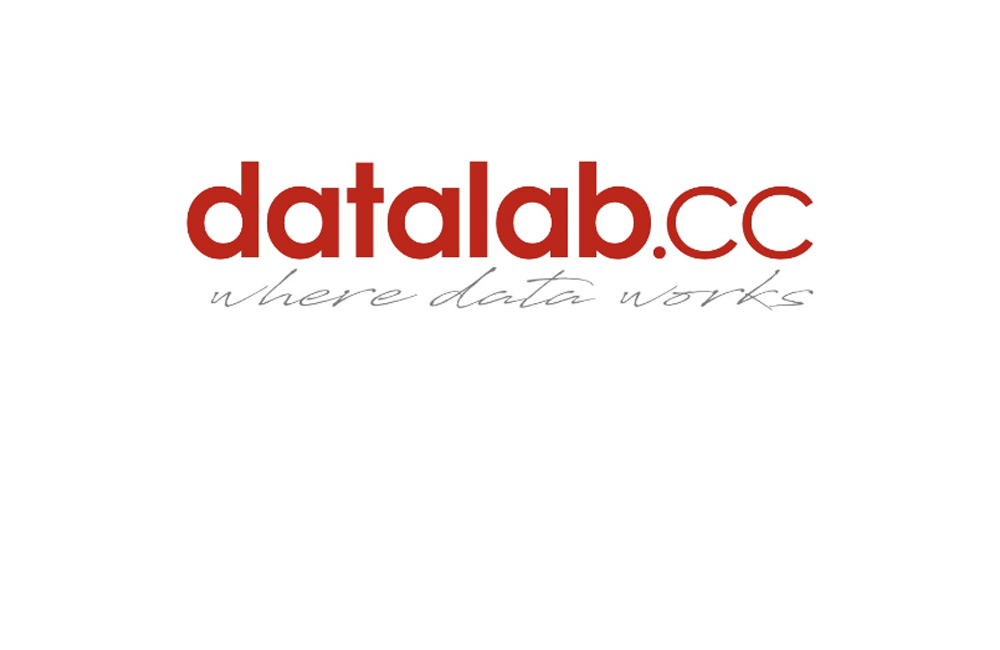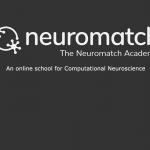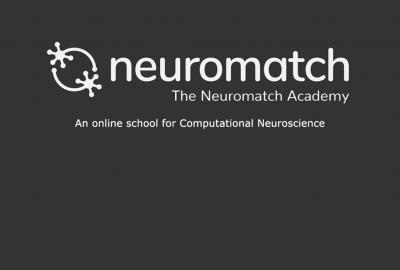Skip to main content# Foundations of Data Science

Category
Level
Beginner

Datalabcc: Foundations of Data Science. Data science relies on several important aspects of mathematics. In this course, you'll learn what forms of mathematics are most useful for data science, and see some worked examples of how math can solve important data science problems.

Off
Course Features
Mathematics for data science practitioners
Primer on elementary algebra
Linear algebra
Calculus
Basic of Probability
Statistics
Lessons of this Course
1
1
Duration:
2:53

Introduction to the Mathematics chapter of Datalabcc's "Foundations in Data Science" series.

2
2
Duration:
3:03

Primer on elementary algebra

3
3
Duration:
5:38

Primer on linear algebra

4
4
Duration:
5:24

Primer on systems of linear equations

5
5
Duration:
4:17

Primer on calculus

6
6
Duration:
8:43

How calculus relates to optimization

7
7
Duration:
5:19

Big O notation

8
8
Duration:
7:33

Basics of probability.

9
9
Duration:
7:57

The probability of a hypothesis, given data.

10
10
Duration:
1:35

Why math is useful in data science.

11
11
Duration:
4:01

Why statistics are useful for data science.

12
12
Duration:
2:23

Statistics is exploring data.

13
13
Duration:
8:01

Graphical data exploration

14
14
Duration:
5:05

Numerical data exploration

15
15
Duration:
10:16

Simple description of statistical data.

16
16
Duration:
4:28

Inferring results from incomplete data

17
17
Duration:
06:04

Basics of hypothesis testing.

18
18
Duration:
08:04

Finding parameter values, confidence intervals.

19
19
Duration:
5:29

Methods for estimating parameters.

20
20
Duration:
3:30

Measuring the correspondece between data and model.

21
21
Duration:
6:15

How to choose useful variables.

22
22
Duration:
5:58

Common problems in statistical modelling.

23
23
Duration:
3:50

Common problems in statistical modelling.

24
24
Duration:
3:18

You don't have to be a wizard to do statistics!

25
25
Duration:
1:43

Overview of possible follow up courses and subjects from the same publisher.

##### Recent courses#### Statistical models#### Machine learning